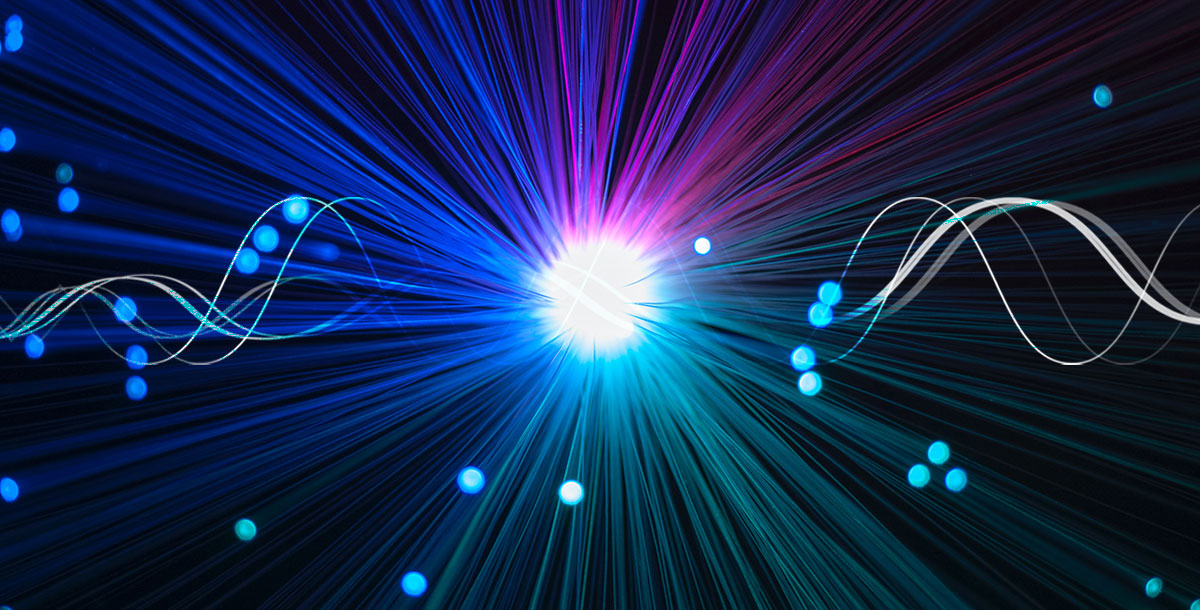# Issue #57 - Simple and Effective Noisy Channel Modeling for Neural MT

24 Oct 2019### Introduction

Neural MT is widely used today and the results are undeniably better compared to the statistical machine translation (SMT) used earlier. One of the core components of an SMT system was the language model. In this post, we will look at how we can benefit from a language model in Neural MT, too. In particular, we will have a quick look at the paper from Yee et. al. (2019)

The probability modeling in SMT is indirect and called noisy channel modeling. In SMT, if our target is y and source is x, to compute target given source P(y|x), we compute source given target P(x|y) and P(y). Here, P(x|y) is called the translation model and P(y) is called the language model. In Neural MT, however, we directly predict P(y|x). We process the whole input sentence with the encoder and feed it to the decoder which generates the target sentence. Now in Neural MT also, we can make use of probability scores from an external language model. However, to do so we also need P(x|y). Yee et. al., (2019) proposed to train a Neural MT system in reverse direction i.e. from target to source to obtain P(x|y). Overall, all probabilities (direct, indirect and language model) are combined as given in the equation below.Where t is the length of the target prefix y, s is the source sentence length and λ1 is a tunable weight. They tested this approach in two scenarios, one with Online Decoding and another for Re-ranking the n-best list. During re-ranking, in the above equation y refers to the full target sentence. They use transformer architecture to train all models.

### Results:

With online decoding setup, they obtain around two BLEU points improvement over the baseline direct model for the German-English language pair. In Re-ranking scenario, they obtain the results as given in the table below. We can see that for all language pairs the BLEU scores with their approach (CH+DIR+LM) are better compared to direct model (DIR).The table below shows the impact of varying beam sizes from 5 to 100 for the German-English language pair. We can see that as we increase the beam size, the quality is also improved with the best result, 43.2 BLEU score obtained for the 100 beam size.### In summary

In summary, from the results, we can say that the approach obtained better results compared to the baseline. However, both online decoding and reranking with large beam size have significant overhead in terms of decoding speed.Author

## Dr. Rohit Gupta

Sr. Machine Translation Scientist